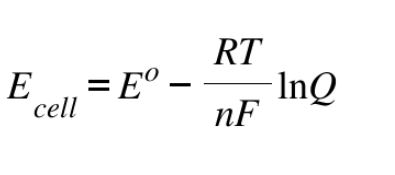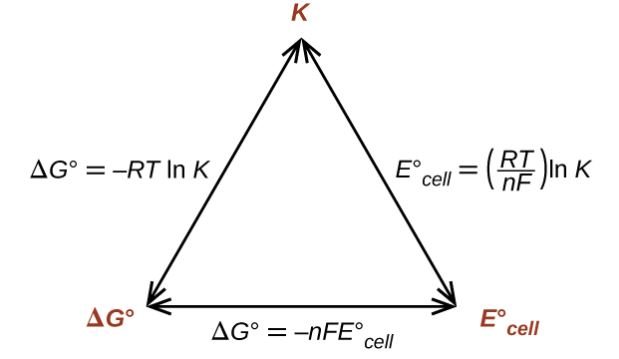# Nernst Equation

## What is the Nernst Equation?

The Nernst equation provides a relation between the cell potential of an electrochemical cell, the standard cell potential, temperature, and the reaction quotient. Even under non-standard conditions, the cell potentials of electrochemical cells can be determined with the help of this equation. The Nernst equation was formulated by (and is named after) the German chemist Walther Hermann Nernst.

The standard cell potential describes the potential difference that arises between two half-cells (having a concentration of 1M) under standard conditions for temperature and pressure (temperature = 273.15K, pressure = 1 atm). However, the cell potential changes with variances in concentration, pressure, and temperature. Since it accounts for this change, the Nernst equation is often used to calculate the cell potential of an electrochemical cell at any given temperature, pressure, and reactant concentration.

### Expression

The expression of the Nernst equation is provided below.Where,

• Ecell is the cell potential of the cell
• E0 is the cell potential under standard conditions
• R is the universal gas constant
• T is the temperature
• n is the number of electrons transferred in the redox reaction
• F is the Faraday constant
• Q is the reaction quotient

### Nernst Equation at a Temperature of 25oC

Generally, the natural logarithm is converted into the common logarithm (log10) in the Nernst equation. At a temperature of 25oC, this equation can be expressed as follows.

$E = E^0 – \frac{0.0592V}{n}log_{10}Q$

As per the Nernst equation, the overall potential of an electrochemical cell is dependent on the reaction quotient. The consumption of the reactants and the formation of products over the course of the reaction causes the cell potential to slowly decrease.

When the reaction reaches chemical equilibrium, the reaction quotient is equal to the equilibrium constant (Kc). At this point, the cell potential of the electrochemical cell is zero (since ΔG = 0 at equilibrium and ΔG = -nFEcell).

## Derivation

The relation between Gibbs energy change (ΔG) and the cell potential (Ecell) is given by the following equation.

ΔG = -nFEcell

Under standard conditions, this equation is transformed as follows.

ΔG0 = -nFE0cell

The Gibbs energy change under non-standard conditions is given by the following equation:

ΔG = ΔG0 + RT lnQ

Substituting the value of ΔG and ΔG0, the equation becomes:

-nFEcell = -nFE0cell + RT lnQ

Dividing the entire equation by ‘-nF’, the equation is transformed as follows.
Ecell = E0cell – (RT/nF) lnQ

By converting the natural logarithm into the base-10 logarithm, the equation can be written as follows.

Ecell = E0cell – (2.303RT/nF) logQ

At standard temperatures (T = 298K), the value of (2.303RT/F) is equal to 0.0592 V. Substituting this value in the previous equation, the following equation can be obtained.

Ecell = E0cell – (0.0592V/n) logQ

Thus, the Nernst equation for the electrochemical cell is derived.

## Determining Equilibrium Constants with the Nernst Equation

When the reactants and the products of the electrochemical cell reach equilibrium, the value of ΔG becomes 0. At this point, the reaction quotient and the equilibrium constant (Kc) are the same. Since ΔG = -nFE, the cell potential at equilibrium is also 0.

Substituting the values of Q and E into the Nernst equation, the following equation is obtained.

0 = E0cell – (RT/nF) lnKc

The relationship between the Nernst equation, the equilibrium constant, and Gibbs energy change is illustrated below.Converting the natural logarithm into base-10 logarithm and substituting T=298K (standard temperature), the equation is transformed as follows.

E0cell = (0.0592V/n) log Kc

Rearranging this equation, the following equation can be obtained.

log Kc = (nE0cell)/0.0592V

Thus, the relationship between the standard cell potential and the equilibrium constant is obtained. When Kc is greater than 1, the value of E0cell will be greater than 0, implying that the equilibrium favors the forward reaction. Similarly, when Kc is less than 1, E0cell will hold a negative value which suggests that the reverse reaction will be favored.

## What are the Applications of the Nernst Equation?

Some important applications of the Nernst equation are listed below.

• Ion concentrations can be determined with the help of this equation.
• This equation is also used in potentiometric titrations.
• In some cases, the pH of a substance can be measured with the help of the Nernst equation.
• The potential of an ion that is moving through a semipermeable membrane can be determined with the help of this equation.

### Limitations

The activity of an ion in a very dilute solution is close to infinity and can, therefore, be expressed in terms of the ion concentration. However, for solutions having very high concentrations, the ion concentration is not equal to the ion activity. In order to use the Nernst equation in such cases, experimental measurements must be conducted to obtain the true activity of the ion.

Another shortcoming of this equation is that it cannot be used to measure cell potential when there is a current flowing through the electrode. This is because the flow of current affects the activity of the ions on the surface of the electrode. Also, additional factors such as resistive loss and overpotential must be considered when there is a current flowing through the electrode.

## Solved Problems

### Example 1

What is the Cell Potential of the Electrochemical Cell in Which the Cell Reaction is:

Pb2+ + Cd → Pb + Cd2+ ; Given that Eocell = 0.277 Volts, temperature = 25oC, [Cd2+] = 0.02M, and [Pb2+] = 0.2M.

#### Solution

Since the temperature is equal to 25oC (~298K), the Nernst equation can be written as follows.

Ecell = E0cell – (0.0592V/n) log10Q

Here, two moles of electrons are transferred in the reaction. Therefore, n = 2. The reaction quotient (Q) is given by [Cd2+]/[Pb2+] = (0.02M)/(0.2M) = 0.1. The equation can now be rewritten as:

Ecell = 0.277 V (Given) – ((0.0592V)/2)*log10(0.1)

Ecell = 0.277V – (0.0296V)(-1) = 0.3066 Volts

Thus, the cell potential of this electrochemial cell at a temperature of 25oC is 0.3066 volts.

### Example 2

The Cu2+ ion concentration in a copper-silver electrochemical cell is 0.1M. If Eo(Ag+/Ag) = 0.8V, Eo(Cu2+/Cu) = 0.34V, and Cell potential (at 25oC) = 0.422V, find the silver ion concentration.

#### Solution

Here, the silver electrode acts as a cathode whereas the copper electrode serves as the anode. This is because the standard electrode potential of the silver electrode is greater than that of the copper electrode. The standard electrode potential of the cell can now be calculated, as shown below.

Eocell = Eocathode – Eoanode = 0.8V – 0.34V = 0.46V

Since the charge on the copper ion is +2 and the charge on the silver ion is +1, the balanced cell reaction is:

2Ag+ + Cu → 2Ag + Cu2+

Since two electrons are transferred in the cell reaction, n = 2. Now, the Nernst equation for this electrochemical cell can be written as follows.

Ecell = E0Cell – (0.0592/2)V*log(0.1/[Ag+]2)

Since log(a/b) = log(a) – log(b) and log(ab) = b*log(a), log(0.1/[Ag+]2) can be rewritten as log(0.1) – 2log[Ag+]. Substituting Ecell = 0.422V and Eocell = 0.46V, the equation becomes:

0.422V = 0.46V – 0.0296V*(-1 – 2log[Ag+]

Therefore, -2log[Ag+] = 1.283 + 1 = 2.283

log[Ag+] = -1.141

[Ag+] = antilog(-1.141) = 0.0722M

Therefore, the silver ion concentration in this copper-silver electrochemical cell is 0.0722M. To learn more about the Nernst equation and other important concepts in electrochemistry, register with BYJU’S and download the mobile application on your smartphone.